# RD Sharma Solutions Class 12 Linear Programming Exercise 30.2

RD Sharma Solutions for Class 12 Maths Chapter 30 Linear Programming Exercise 30.2, is provided here. Students who wish to secure an excellent score then solving RD Sharma Solutions Class 12 is a must. These solutions will also help you in obtaining knowledge and strong command over the subject. Students can refer and download the pdf which is readily available from the links given below.

## Download PDF of RD Sharma Solutions for Class 12 Maths Chapter 30 Exercise 2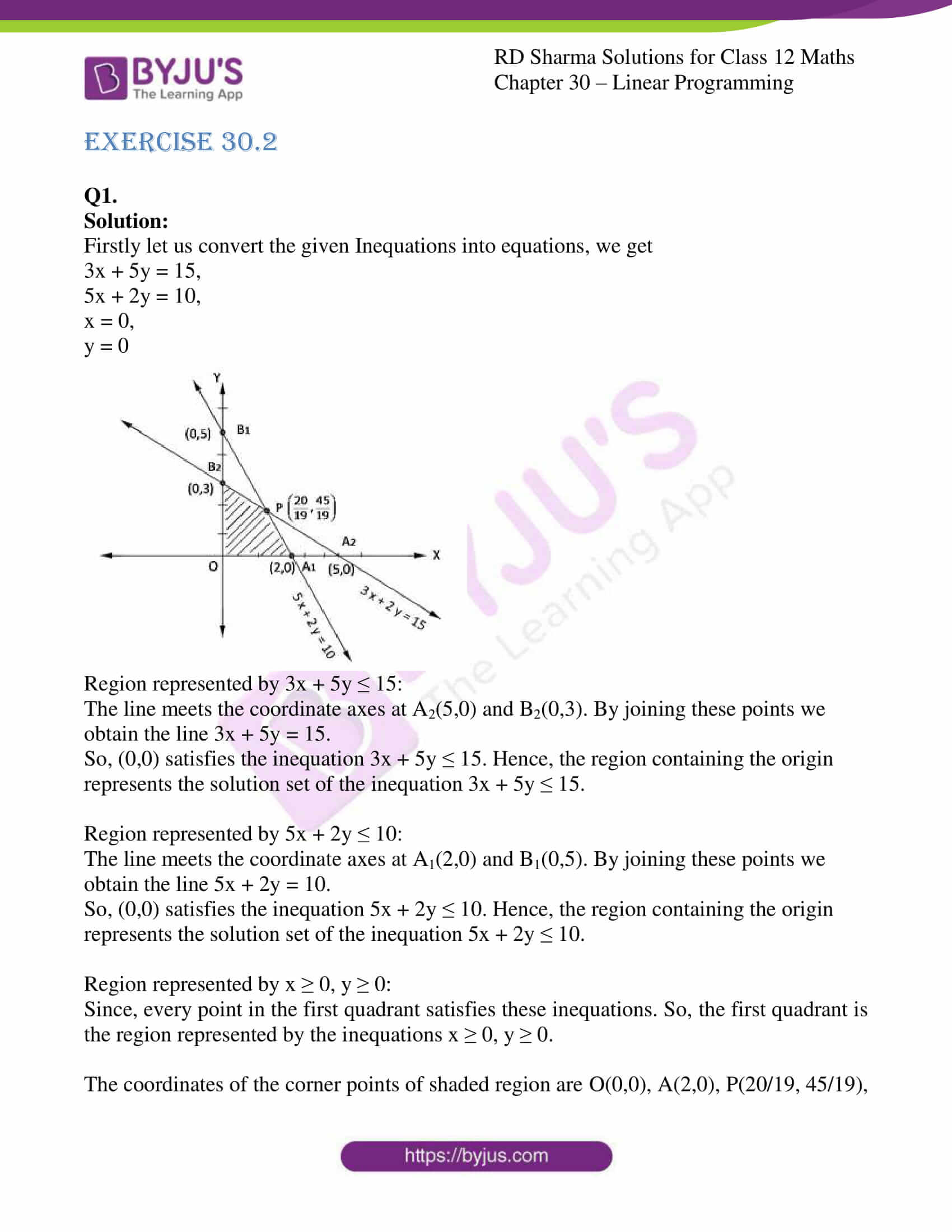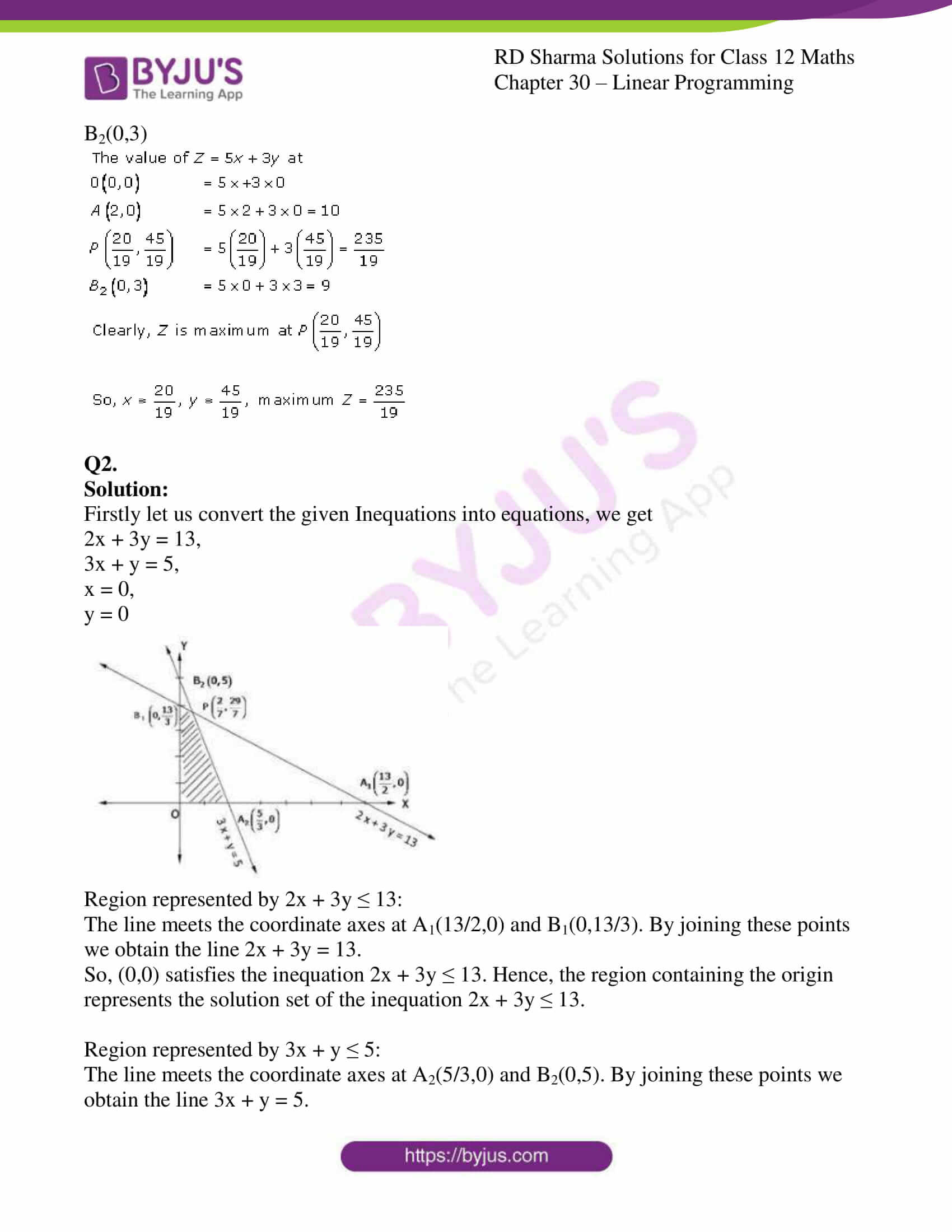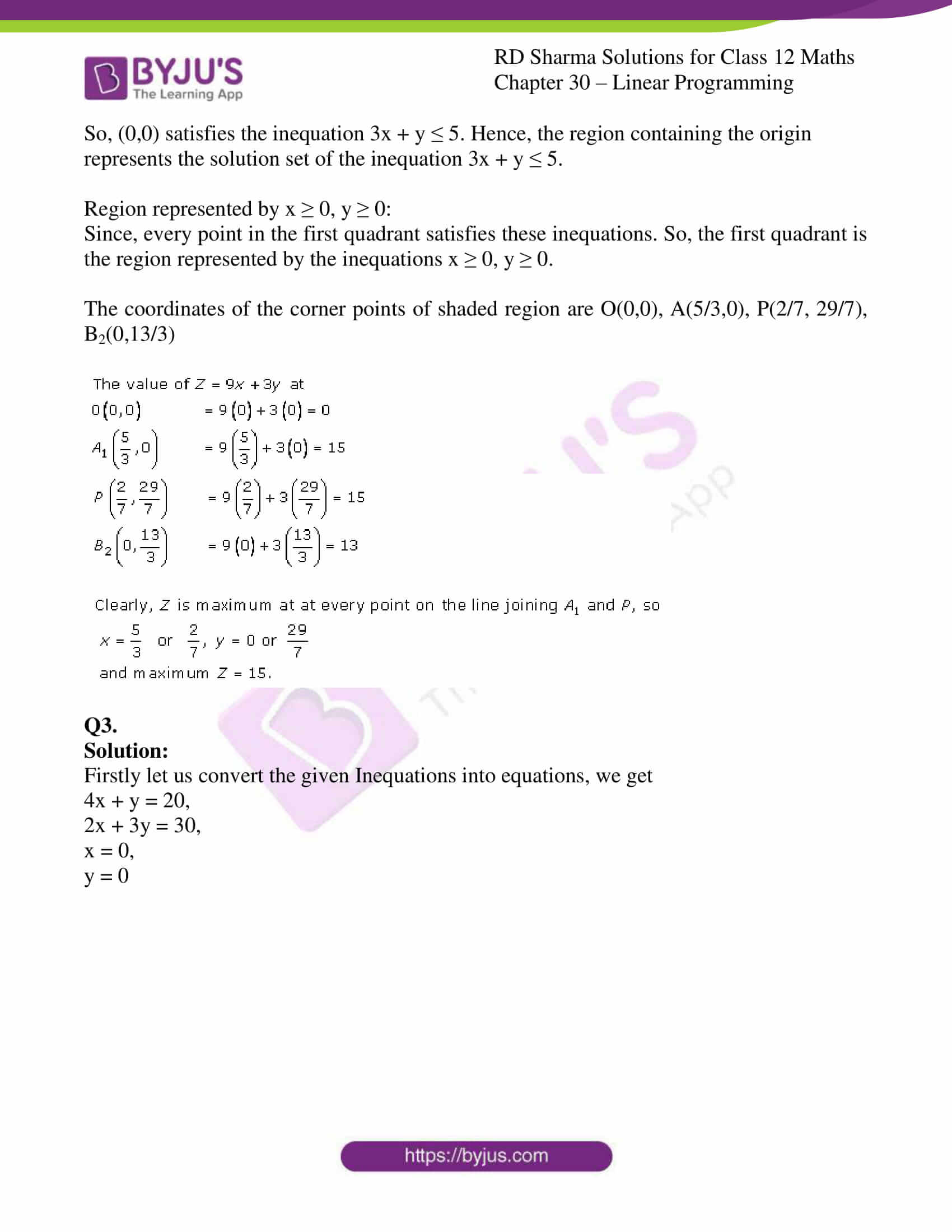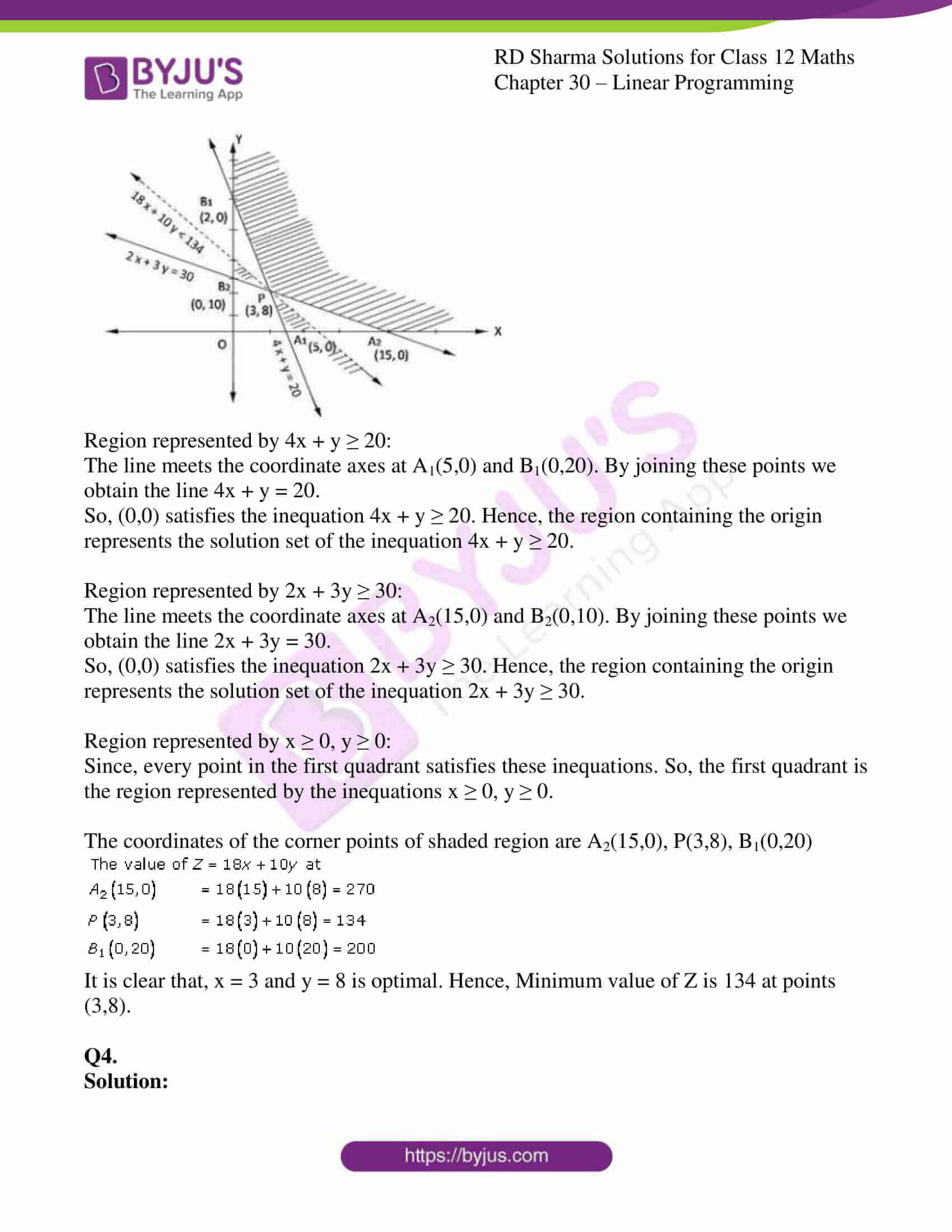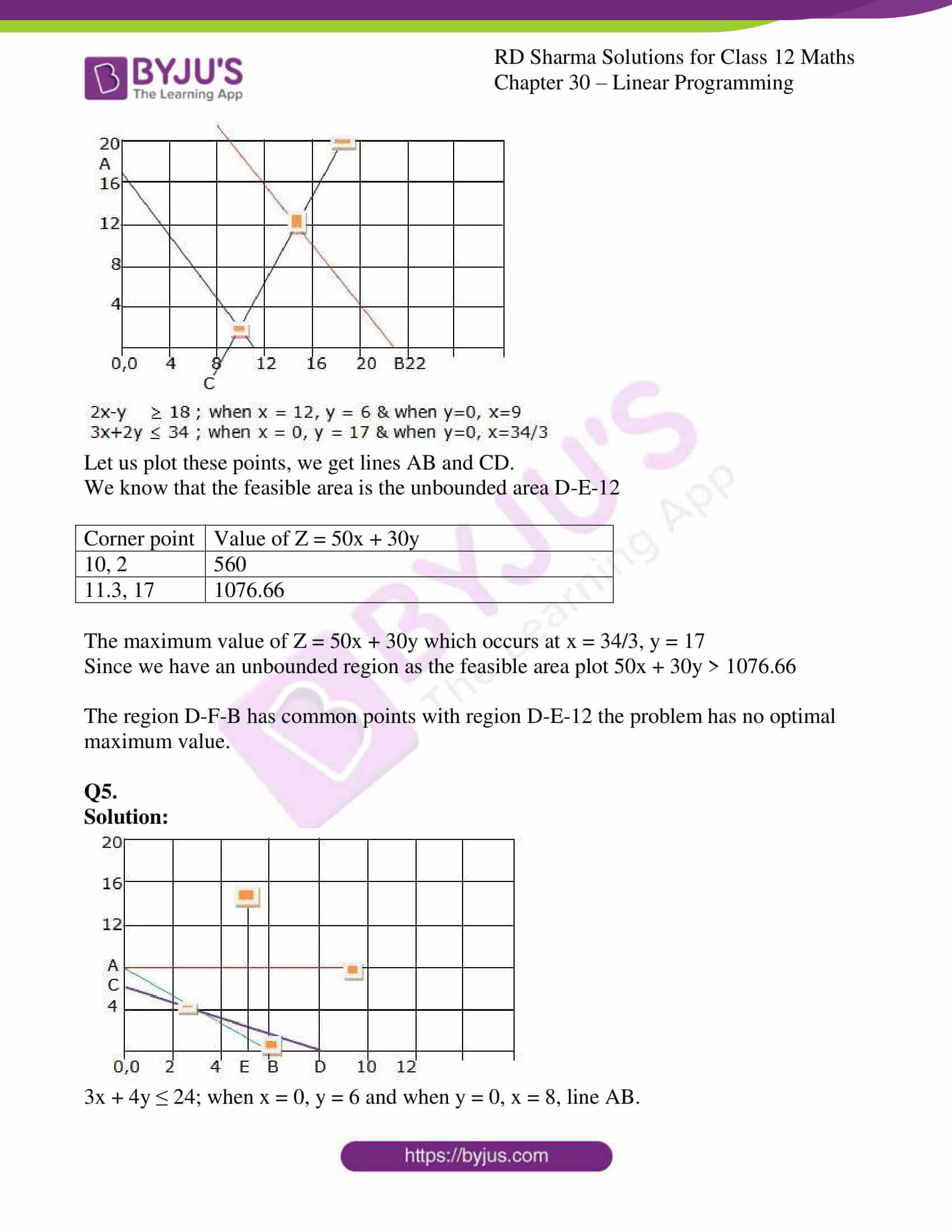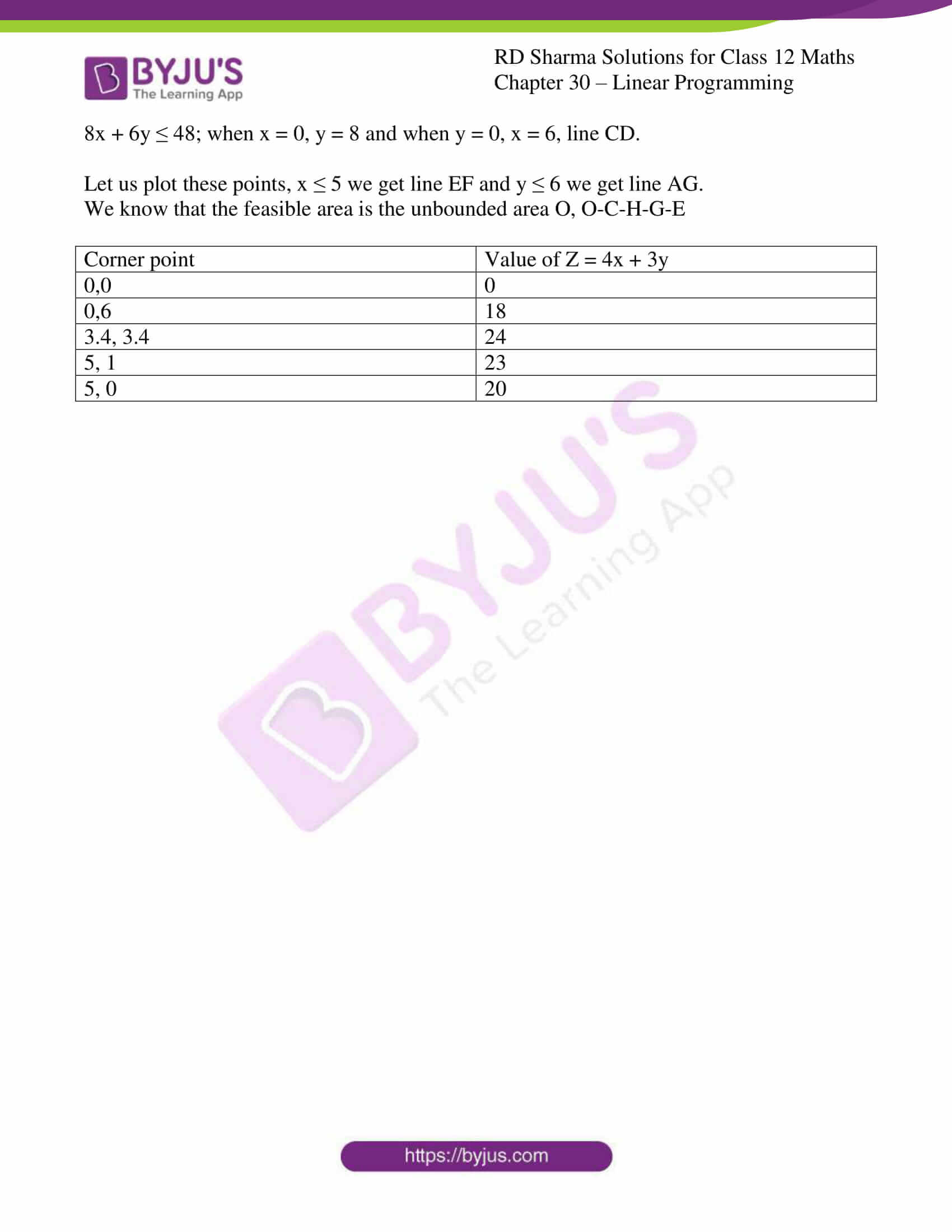### Access RD Sharma Solutions for Class 12 Maths Chapter 30 Exercise 2

EXERCISE 30.2

Q1.

Solution:

Firstly let us convert the given Inequations into equations, we get

3x + 5y = 15,

5x + 2y = 10,

x = 0,

y = 0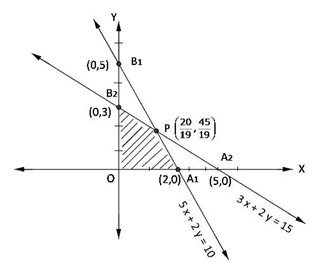Region represented by 3x + 5y ≤ 15:

The line meets the coordinate axes at A2(5,0) and B2(0,3). By joining these points we obtain the line 3x + 5y = 15.

So, (0,0) satisfies the inequation 3x + 5y ≤ 15. Hence, the region containing the origin represents the solution set of the inequation 3x + 5y ≤ 15.

Region represented by 5x + 2y ≤ 10:

The line meets the coordinate axes at A1(2,0) and B1(0,5). By joining these points we obtain the line 5x + 2y = 10.

So, (0,0) satisfies the inequation 5x + 2y ≤ 10. Hence, the region containing the origin represents the solution set of the inequation 5x + 2y ≤ 10.

Region represented by x ≥ 0, y ≥ 0:

Since, every point in the first quadrant satisfies these inequations. So, the first quadrant is the region represented by the inequations x ≥ 0, y ≥ 0.

The coordinates of the corner points of shaded region are O(0,0), A(2,0), P(20/19, 45/19), B2(0,3)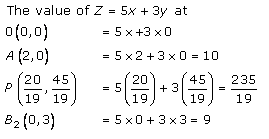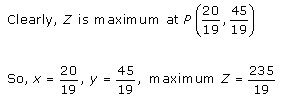Q2.

Solution:

Firstly let us convert the given Inequations into equations, we get

2x + 3y = 13,

3x + y = 5,

x = 0,

y = 0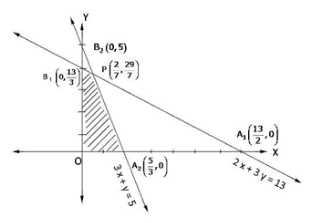Region represented by 2x + 3y ≤ 13:

The line meets the coordinate axes at A1(13/2,0) and B1(0,13/3). By joining these points we obtain the line 2x + 3y = 13.

So, (0,0) satisfies the inequation 2x + 3y ≤ 13. Hence, the region containing the origin represents the solution set of the inequation 2x + 3y ≤ 13.

Region represented by 3x + y ≤ 5:

The line meets the coordinate axes at A2(5/3,0) and B2(0,5). By joining these points we obtain the line 3x + y = 5.

So, (0,0) satisfies the inequation 3x + y ≤ 5. Hence, the region containing the origin represents the solution set of the inequation 3x + y ≤ 5.

Region represented by x ≥ 0, y ≥ 0:

Since, every point in the first quadrant satisfies these inequations. So, the first quadrant is the region represented by the inequations x ≥ 0, y ≥ 0.

The coordinates of the corner points of shaded region are O(0,0), A(5/3,0), P(2/7, 29/7), B2(0,13/3)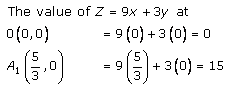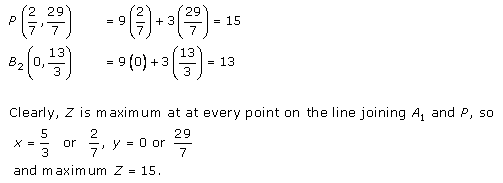Q3.

Solution:

Firstly let us convert the given Inequations into equations, we get

4x + y = 20,

2x + 3y = 30,

x = 0,

y = 0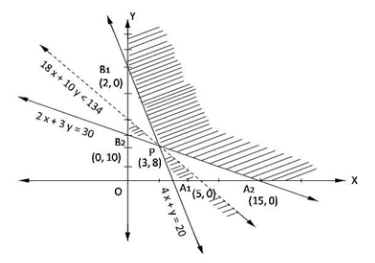Region represented by 4x + y ≥ 20:

The line meets the coordinate axes at A1(5,0) and B1(0,20). By joining these points we obtain the line 4x + y = 20.

So, (0,0) satisfies the inequation 4x + y ≥ 20. Hence, the region containing the origin represents the solution set of the inequation 4x + y ≥ 20.

Region represented by 2x + 3y ≥ 30:

The line meets the coordinate axes at A2(15,0) and B2(0,10). By joining these points we obtain the line 2x + 3y = 30.

So, (0,0) satisfies the inequation 2x + 3y ≥ 30. Hence, the region containing the origin represents the solution set of the inequation 2x + 3y ≥ 30.

Region represented by x ≥ 0, y ≥ 0:

Since, every point in the first quadrant satisfies these inequations. So, the first quadrant is the region represented by the inequations x ≥ 0, y ≥ 0.

The coordinates of the corner points of shaded region are A2(15,0), P(3,8), B1(0,20)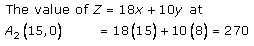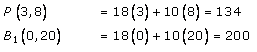It is clear that, x = 3 and y = 8 is optimal. Hence, Minimum value of Z is 134 at points (3,8).

Q4.

Solution: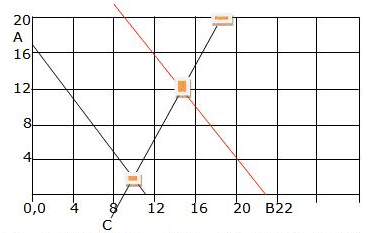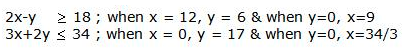Let us plot these points, we get lines AB and CD.

We know that the feasible area is the unbounded area D-E-12

 Corner point Value of Z = 50x + 30y 10, 2 560 11.3, 17 1076.66

The maximum value of Z = 50x + 30y which occurs at x = 34/3, y = 17

Since we have an unbounded region as the feasible area plot 50x + 30y > 1076.66

The region D-F-B has common points with region D-E-12 the problem has no optimal maximum value.

Q5.

Solution: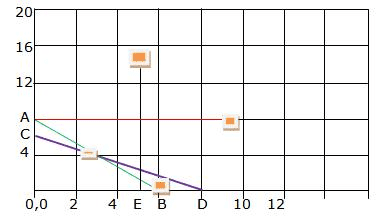3x + 4y ≤ 24; when x = 0, y = 6 and when y = 0, x = 8, line AB.

8x + 6y ≤ 48; when x = 0, y = 8 and when y = 0, x = 6, line CD.

Let us plot these points, x ≤ 5 we get line EF and y ≤ 6 we get line AG.

We know that the feasible area is the unbounded area O, O-C-H-G-E

 Corner point Value of Z = 4x + 3y 0,0 0 0,6 18 3.4, 3.4 24 5, 1 23 5, 0 20